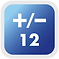# K2-K3 SyllabusNumbers up to 10

Recognize numbers up to 10 through counting, reading, and writing

Learn how to count in ascending and descending order

Understand odd and even numbers

Compare two sets of objects using a one-to-one relationship

Understand the basic combination of numbers: 1-10Numbers up to 20

Recognize numbers up to 20 through counting, reading, and writing

Learn ordinal and cardinal numbers

Understand the basic combination of numbers: 11-18Numbers up to 100

Recognize numbers up to 100 through counting, reading, and writing

Learn the basic concepts of the ones and tens digits

Learn how to count by 2, 5, and 10

Understand the basic combination of numbers: 1-100Basic Addition and Subtraction

Understand the basic concept of addition and subtraction

Record addition and subtraction with numbers up to 18 using horizontal form

Learn the concept of zero through subtraction

Understand the relationship between addition and subtraction

Use practical examples to understand the commutative property of additionAddition & Subtraction (1)

Add up to two digits, including learning how to carry digits over

Learn how to calculate using vertical form

Add three numbers in succession

Subtract up to two digits, excluding decomposition subtraction

Solve simple word problemsCommon Currency (1)

Identity currency that is in circulation in Hong Kong

Learn how to read the price tag of items

Learn to use money through activities

Solve simple application questionsTime (1)

Understand what “Hour” is

Learn how to tell the time by the Hour

Know the names of the days in a week

Know that there are twelve months in a year

Read out the year, month, day, and week in the calendarLength and Distance

Learn length and distance

Directly compare the length and the distance between the objects

Choose the appropriate units for measurement3D Shapes (1)

Understand the properties of 3-D shapes

Intuitively distinguish various 3-D shapes

Categorize 3D shapes like cylinders, cones, and spheresStraight Lines and Curved Lines

Understand straight and curved lines

Intuitively distinguish various straight and curved lines

Use different ways to draw straight and curve lines

# Advanced K2-K3 SyllabusThree Digit Numbers

Recognize three digits through counting, reading, and writing

Recognize the unit value of the hundreds digit

Learn to count by 50 and 100

Estimate the number of objectsFour Digit Numbers

Recognize four digits through counting, reading, and writing

Recognize the unit value of the thousands digit

Learn to count by 500 and 1000Fractions (1)

Recognize the fraction as a whole and a part of a group of objects

Understand the relationship between a fraction a 1

Compare the size of fractions with the same denominator or the same numerator

Solve simple word problemsBasic Multiplication

Understand the concept of multiplication

Learn how to write the multiplication tables (1-10)

Perform basic multiplication operations

Understand multiplication's exchange properties through practical examples

Solve simple application problems